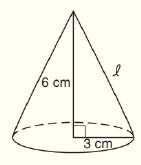Chapter 9.CT, Problem 11CTElementary Geometry For College St...

7th Edition
Alexander + 2 others
ISBN: 9781337614085

Solutions

Chapter
SectionElementary Geometry For College St...

7th Edition
Alexander + 2 others
ISBN: 9781337614085
Textbook Problem

Find the slant height of the right circular cone below. Leave the answer in simplified radical form.To determine

To find:

The slant height of right circular cone.

Explanation

Given:

The right circular cone with indicated measurements is shown below.

Calculation:

In a right circular cone, l2=r2+h2

From, the figure we see that r=3 cm, h=6 cm.

Substitute the known values in l2=r2+h2

Still sussing out bartleby?

Check out a sample textbook solution.

See a sample solution

The Solution to Your Study Problems

Bartleby provides explanations to thousands of textbook problems written by our experts, many with advanced degrees!

Get Started

Find more solutions based on key concepts